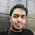## Wednesday, May 5, 2010

### My Day with Eclipse MAT and Dominator Tree

Eclipse MAT (Memory Analyzing Tool) is a feature rich Heap Dump analysis tool. This helps you to track down important pointers on MOB (Memory Out of Bound) Exception. Sun JVM (1.4.2 onwards) supports the feature of creating HPROF HeapDump. MAT parses heap dump file (i.e. .hprof) and gives graphics intuitive description of Memory Leak suspects.

The most important information of Heap Dump is “Dominator Tree”, in simple word, it lists the objects and the amount of memory space that they are holding. Before understanding “Dominator Tree”, you need to know 3 important concepts of Java Objects- Shallow Size, Retain Size and Retain Set. There is a nice article which explains this concept.

How to find out the Biggest Retain Set ?

The best possible approach to find out the biggest “Retain Set” and hence the maximum prospect of releasing heap storage on next Garbage Collection (GC), is “Dominator Tree” graph. Dominator Tree Algorithm converts the Object Reference Graph to Dominator Tree, the fastest way to find out the biggest “Retain Set”.

In the following section I'll try to explain how to analyze Dominator Tree with an example code where I have added multiple inter-dependent object references and an infinite loop to get MOB Exception.

Dominator Tree (Eclipse MAT)Dominator Tree (java_pid1076.hprof)

Retained Heap = 96 (Shallow Heap of Data i.e. 32 + Retained Heap of A, B, C, D i.e. 48)

Note:You need to set VM argument (-XX:+HeapDumpOnOutOfMemoryError) to get HPROF heap dump if there is any MOB Exception.

Object Reference graph to Dominator Tree ConversionObject Reference Graph

Data-> {a, b, c, d}

b-> {c}

d-> {b, c}

Dominator Tree (Retain Set)

Data-> {Data, a, b, c, d}

a -> {a}

b -> {b}

c -> {c}

d -> {d}

Note: Here a, b, c, d are instances/objects of A, B, C and D classes.

Above example uses following classes-

public class HeapDemo {

public void eatUpMemo(){

// do some

ArrayList fatMan = new ArrayList();

for(;;){

Data d = new Data();

}

}

}

public class Data {

String title = "ABC";

A a = new A();

B b = new B();

C c = b.getC();

D d = new D(b);

}

public class A {

int x = 10;

}

public class B {

int y = 20;

C c = new C();

public C getC(){

return c;

}

}

public class C {

int z = 30;

}

public class D {

C c;

B b;

public D(B b){

this.b = b;

c = b.getC();

}

}

Reference:

1.2.